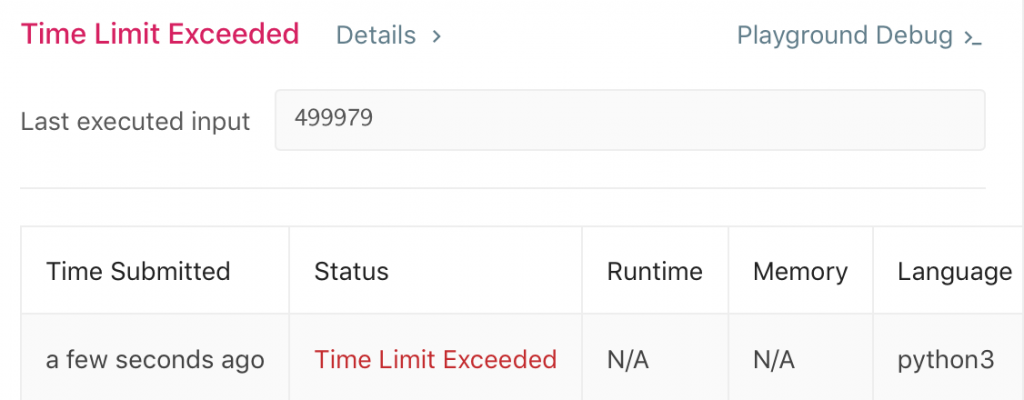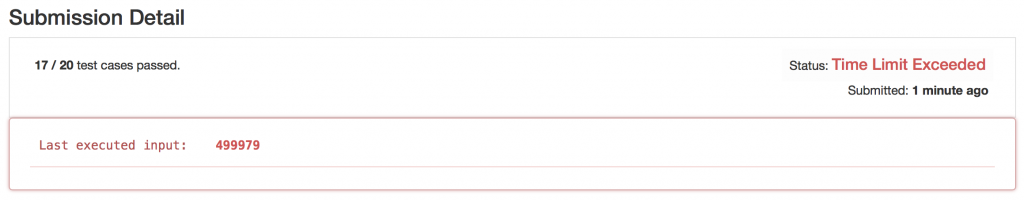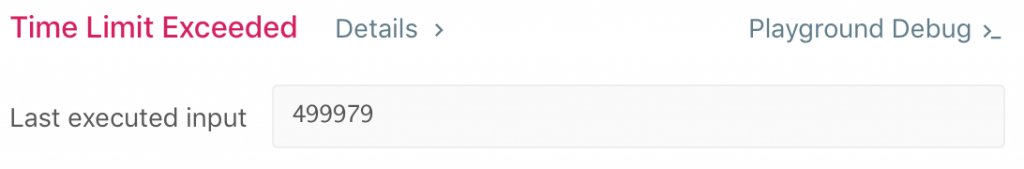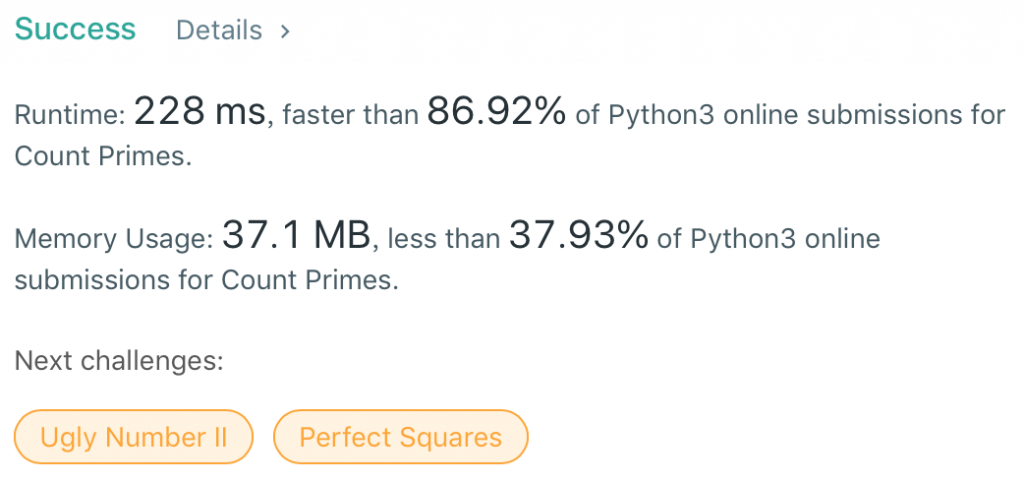#DAY 30
0

## #204 Count Primes

### 進入正題

Count the number of prime numbers less than a non-negative number, n.
Example:
Input: 10
Output: 4
Explanation: There are 4 prime numbers less than 10, they are 2, 3, 5, 7.

``````def countPrimes(self, n: int) -> int:
count = 0
for num in range(n):
if num > 1:
for i in range(2,num):
if (num % i) == 0:
break
else:
count = count + 1
return count
````````````def countPrimes(self, n: int) -> int:
def func_get_prime(num):
return filter(lambda x: not [x%i for i in range(2, int(math.sqrt(x))+1) if x%i ==0], range(2,num))

return len(list(func_get_prime(n)))
``````

Submit後還是超過時間：``````def countPrimes(self, n: int) -> int:
if n < 3:
return 0
primes = [True] * n
primes = primes = False
for i in range(2, int(n ** 0.5) + 1):
if primes[i]:
primes[i * i: n: i] = [False] * len(primes[i * i: n: i])
return sum(primes)
``````

Submit後速度就及格了，最後結果如下：### 參考資料

Leetcode新手挑戰30天31